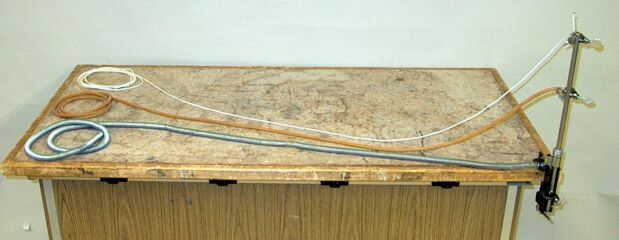You can compare the speed of a traveling wave on a spring, rubber tubing and nylon cord by stretching and plucking each one.

This demonstration allows you to show some of the properties of a one-dimensional transverse wave. That is, a wave that is traveling along a line (as opposed to propagating over a surface or through a volume), and for which the displacement of the medium is perpendicular to the direction in which the wave travels. By holding the free end of one of the media and pulling it taught, then displacing the medium with your other hand and suddenly letting go (or plucking it), you send a pulse towards the opposite end of the medium. Since this end is fixed, the reflected pulse is inverted.

An important characteristic of a wave is its speed in the medium. This speed depends on the elasticity of the medium, which provides the restoring force that brings the displaced section back to its original position, and the inertia of the displaced section, which is determined in a linear medium by its mass per unit length, μ. We take the elasticity to be the tension on the medium, T. We can imagine a pulse traveling down a string, and further imagine that a small section of string at the top of the pulse, of length Δl, forms an arc along a circle whose radius is R. This arc subtends an angle, θ, to either side of the vertical. The mass of this section of string is μ Δl, and the tension is the tangential pull of the string on either side. The horizontal components of the tension are equal and opposite, so they cancel, and the vertical components, which equal T sin θ, add, so that the total restoring force is 2T sin θ. Since we are considering a very short length of string, θ is small, so sin θθ and 2T sin θ = 2 = 2Tl/2)/R, or TΔl/R. The section of string is traveling around the circle, and the centripetal force accelerating it towards the center of the circle is thus TΔl/R.

The centripetal force on our section of string also has to equal μ Δl v2/R, where v is the tangential velocity of the section of string. At the top of the circle, v is horizontal and equals the speed of the pulse traveling along the cord. Thus, we have TΔl/R = μ Δl v2/R, which we can rearrange to v = √(T/μ).

We can see from this that the higher the tension, the greater the wave speed, and the denser the medium, that is, the greater its mass per unit length, the lower the wave speed. For the cord, tubing and spring in this demonstration, with no applied tension, μ is approximately 6.0 × 10-3 kg/m, 3.6 × 10-2 kg/m and 0.438 kg/m, respectively.

References:

1) Resnick, Robert and Halliday, David. Physics, Part One, Third Edition (New York: John Wiley and Sons, 1977), pp. 412-413.